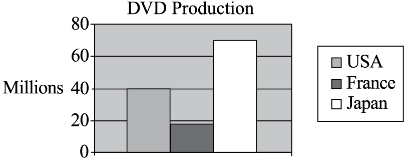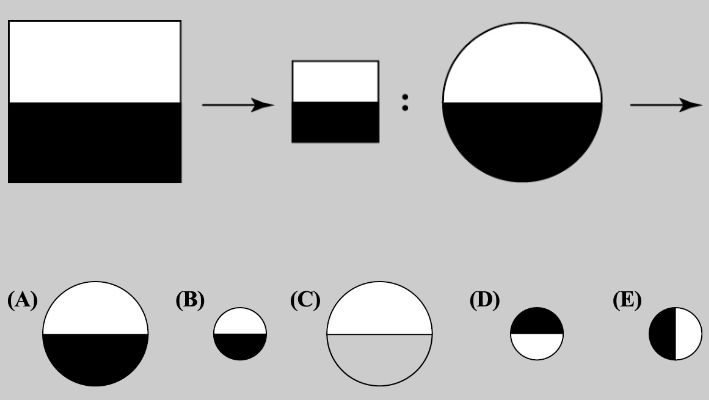# TACHS CHSEE Math and Ability Practice Test 2023

## TACHS CHSEE Math Practice Test 2023

The Math section of TACHS measures your ability to solve math problems in a variety of ways. For each math question, you will be given answer choices from which to choose. The first portion tests your knowledge of math concepts such as fractions, factors, multiples, multiplication, division, and decimals.

The second portion of this section tests your ability to solve word problems using the basic math concepts mentioned above.

The third portion of this section measures your ability to estimate, or do the math in your head, without using a pencil and paper.

The closest estimate of 3.9 × 4.1 is ______.

(A) 9
(B) 12
(C) 16
(D) 20

The correct answer is (C). 3.9 × 4.1 ≈ 16

The fourth portion of this section measures your ability to interpret data, or to work with charts and graphs.The chart above shows the annual production of DVDs in various countries as measured in millions. Based on the information in the chart, about how many DVDs does Japan produce each year?

(A) 70
(B) 70,000,000
(C) 60
(D) 60,000,000

The correct answer is (B). Japan is represented by the white bar that places yearly DVD production at 70 million.

TACHS CHSEE Ability Practice Test

The Ability section of the exam tests your abstract reasoning ability. You will be presented with visual tasks that require you to generalize from one item or series of items to another. These are reasoning skills that are going to be tested, not academic abilities, so don’t worry if this sounds unlike anything you’ve been taught in school. Identifying patterns and looking ahead for the logical outcome of series of changes to shapes is all that will be required of you in this section.The correct answer is (B). The first pair share a similar item, but the second rectangle is smaller than the first. The same logic must hold true for the second pair. Choice (B) is a smaller version of the black-and-white circle in the series above.